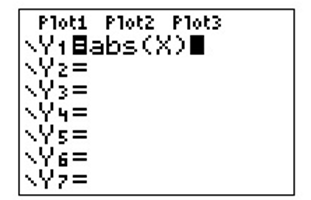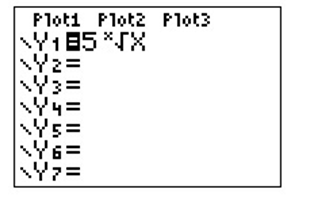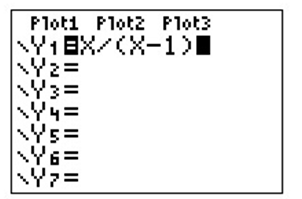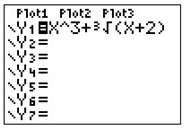# Plot a graph.### Precalculus: Mathematics for Calcu...

6th Edition
Stewart + 5 others
Publisher: Cengage Learning
ISBN: 9780840068071### Precalculus: Mathematics for Calcu...

6th Edition
Stewart + 5 others
Publisher: Cengage Learning
ISBN: 9780840068071

#### Solutions

Chapter 1.9, Problem 76E

a.

To determine

## Plot a graph.

Expert Solution### Explanation of Solution

Calculation:

Consider the equation y=|x| .

Enter y=|x| , we have to enter the function using the absolute value function of the calculator.

The display is shown below:b.

To determine

### Plot a graph.

Expert Solution### Explanation of Solution

Calculation:

Consider the equation y=x5

Enter y=x5 , we need to enter the function using the nth root function of the calculator.

The display is shown below:c.

To determine

### Plot a graph.

Expert Solution### Explanation of Solution

Calculation:

Consider the equation y=xx1

Enter y=xx1 , we have to enter the function as y=xx1 .

Note that if we miss putting the parentheses around the factor x1 , the calculator will plot the line y=0 instead of the function y=xx1 .

The display is shown below:d.

To determine

### Plot a graph.

Expert Solution### Explanation of Solution

Calculation:

Consider the equation y=x3+x+23

Enter y=x3+x+23 , we have to enter the function using the cube root function of the graphing calculator.

The display is shown below:### Have a homework question?

Subscribe to bartleby learn! Ask subject matter experts 30 homework questions each month. Plus, you’ll have access to millions of step-by-step textbook answers!